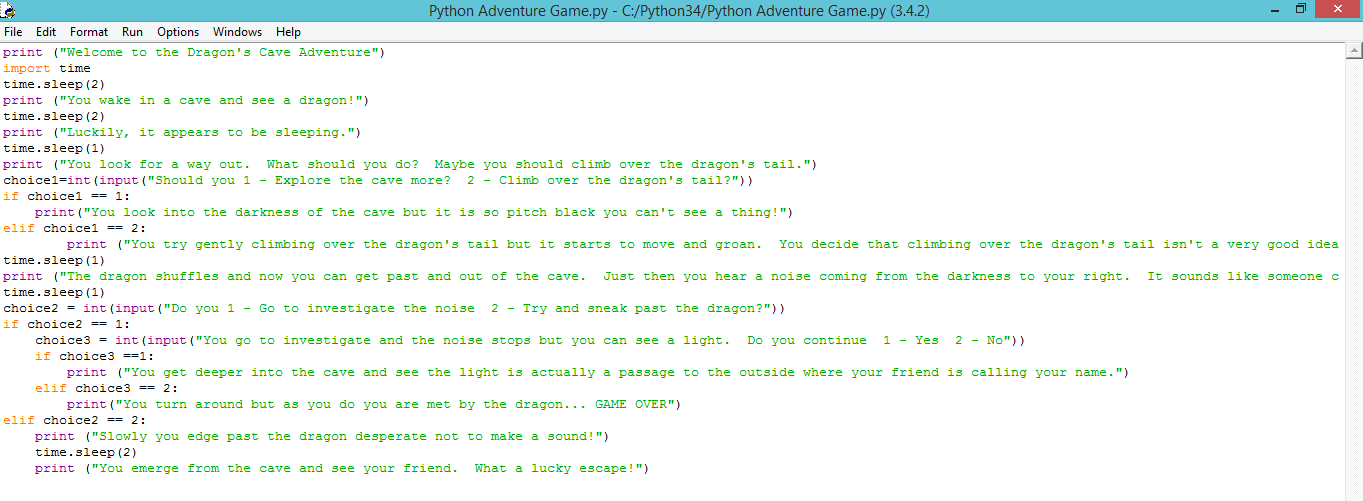## 21 Jan 2020  Python Basics

### 1.1 Complete activities below from Intro to While Loop

Skip these units

casting,Lists, Tuples ,Sets and Dictionaries

### 1.3 Using the table below try and complete 1 - 4

Try to complete the following exercises; the table above will help you:

1. Write a program that will ask the user for three integer numbers and then add these numbers
together before displaying the answer. The input and output should be user friendly.

2. Write a program that will ask the user for four integer numbers and then add them together
and then divide that total by 4 to show the average. The input and output should be user
friendly.

3. Write a program that will ask for the total bill at the restaurant and then the number of diners
who ate that meal. It will then work out how much each person should pay for an equal share
of the meal.

4. Write a program that will ask the user the height and then the width of a rectangle and which
will then show the area of the rectangle.

## Lesson 1 Input Statements and if Statements

### Lesson 1.1 Input Statements

#### WALT: Use input statements in Python

PART 1

Independent work: Test your program with a partner :

Coding challenge 1: Write a program that asks the user to enter how many children are in their class and how many exercise books each child has. The program should then output how many exercise books there are altogether.

Coding challenge 2: Write a program which asks the user their name and how many days until their next birthday. The program should then output a personalized sentence with a rough approximation of the number of seconds until their next birthday.

### Lesson 1.2 Conditional Statements

Complete the program below

Program Challenge in Python

Create Program "Find The Skeleton"

Scenario 4 rooms and 2 attempts to find the skeleton

If the user selects the wrong room, inform them how many tries left and also give them a clue.

## Lesson 2 Arithmetic Operators

Try to complete the following exercises; the table above will help you:

1. Write a program that will ask the user for three integer numbers and then add these numbers
together before displaying the answer. The input and output should be user friendly.

2. Write a program that will ask the user for four integer numbers and then add them together
and then divide that total by 4 to show the average. The input and output should be user
friendly.

3. Write a program that will ask for the total bill at the restaurant and then the number of diners
who ate that meal. It will then work out how much each person should pay for an equal share
of the meal.

4. Write a program that will ask the user the height and then the width of a rectangle and which
will then show the area of the rectangle.

5. Alter the previous program so that it will ask for the height, width and also the depth of a box
and then show the total volume of that box.

6. Write a program which will ask for the height, width and depth of a small box and then the
height, width and depth of a larger box. Work out how much space is remaining around the
small box when it is placed inside the bigger box.

7. Write a program that will ask the user for how many sweets are in the bag. It should also ask
how many people the sweets should be shared between. Use the whole number division to
work out how many sweets each person should have and use the remainder division to find out
how many sweets would be left remaining. For example if there are 14 sweets in the bag and
this was to be divided between 3 people then the programme should display “There will be 4
sweets each and there would be 2 left over.”

## Activity Text Based Adventure GameIndependent work: